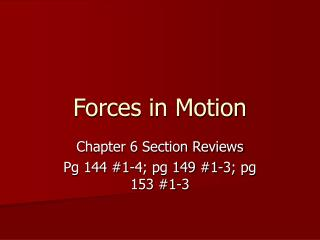DownloadDownload PresentationForces in Motion

# Forces in Motion

Télécharger la présentation## Forces in Motion

- - - - - - - - - - - - - - - - - - - - - - - - - - - E N D - - - - - - - - - - - - - - - - - - - - - - - - - - -
##### Presentation Transcript

1. Forces in Motion Chapter 6 Section Reviews Pg 144 #1-4; pg 149 #1-3; pg 153 #1-3

2. Pg 144 #1-4 1. How does air resistance affect the acceleration of falling objects? Air resistance slows or stops acceleration of falling objects. 2. Explain why an astronaut in an orbiting spaceship floats. An astronaut in an orbiting spaceship floats because both the astronaut and the spaceship are in free fall. Since both fall at the same rate, the astronaut floats inside. The astronaut has not sensation of falling.

3. Pg 144 #1-4 (cont.) 3. How is an orbit formed? An orbit is formed by combining two motions: a forward motion and free fall toward Earth. The path that results is a curve that matches the curve of Earth’s surface. 4. Think about a sport you play that involves a ball. Identify at least four different instances in which an object is in projectile motion. Basketball – a player jumping to dunk the ball, a ball passed from one player to another, a ball shot toward the basket, a ball bounced on the floor.

4. Pg 149 #1-3 1. How is inertia related to Newton’s law of motion? Newton’s first law says that matter resists any change in motion. Inertia is the tendency of objects (matter) to resist changes in motion. Newton’s first law is also known as the law of inertia. 2. Name two ways to increase the acceleration of an object. You can increase the acceleration of an object by increasing the force causing the acceleration or by reducing the object’s mass.

5. Pg 149 #1-3 (cont.) 3. If the acceleration due to gravity were somehow doubled to 19.6 m/s/s, what would happen to your weight? If the acceleration due to gravity were doubled, your weight would double. This is because of Newton’s second law: F = ma. Weight is the force due to the acceleration on mass. If acceleration is doubled and mass remains the same, the force (weight) is doubled, too.

6. Pg 153 #1-3 1. Name three action and reaction force pairs involved in doing your homework. Name what object is exerting and what object is receiving the forces. Using a pencil or pen (action: hand pushing on pencil; reaction: pencil pushing back on hand OR action: pencil pushing on paper; reaction: paper pushing on pencil).

7. Pg 153 #1-3 (cont.) 2. Which has more momentum, a mouse running at 1 m/s north or an elephant walking at 3 m/s east? Explain your answer. The elephant has both a greater mass and greater velocity.

8. Pg 153 #1-3 (cont.) 3. When a truck pulls a trailer, the trailer and truck accelerate forward even though the action and reaction forces are the same size but in opposite directions. Why don’t these forces balance each other out? The action and reaction forces do not balance each other because the forces are acting on two different objects. Because they act on two different objects, you cannot combine them to determine a net force.